Limits: by Factoring
 Home > Lessons > Limits: by Factoring Search | Updated May 25th, 2021
Introduction

This lesson page will inform you how to find limits of certain rational functions using polynomial factors. Here are the sections within this page:

If your factoring and reducing of rational expression skills need assistance, review these lessons before proceeding.esson: Factoringesson: Rational Expressions

 Using Substitution For some limits, the problem can be solved using substitution. Here is an example of such a limit.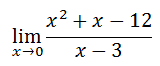We need only replace the xĺs with 0, like so.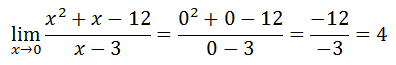Here are two more examples.However, not all problems are so simple. The next section will show how substitution alone is not always a reliable technique for calculating a limit.
 Using Factors In the last section, we saw how we could use substitution for this problem.Now, letĺs see what happens if we use substitution as we approach x = 3 instead of x = 0.This is a call for mathematical alarm because the denominator cannot be equal to zero. Why? This video explains why division by zero is illogical. https://www.youtube.com/watch?v=3hkFl4yYM_I     Anytime we substitute a value into a function and get a denominator equal to zero, we get an undetermined result. It means the process tells us absolutely nothing about the limit. It also means we have to try some other strategy.     The other strategy is to factor the polynomial.     If we factor the function, we can reduce the rational function, like so.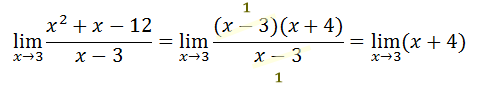Now we can use substitution because we no longer get a zero in the denominator when we do so.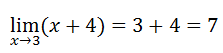Here are two more examples using factoring as a strategy.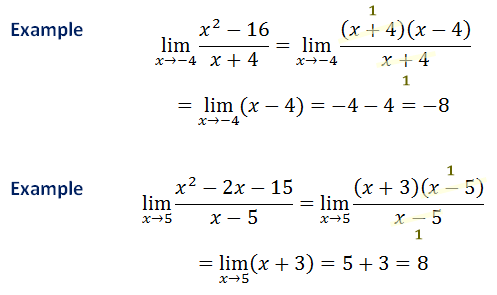ideo: Limits: By Factoringuiz: Limits: By Factoring Instructional Videos Try this instructional video to learn this lesson.ideo: Limits: By Factoring Interactive Quizmasters Try this interactive quizmaster to determine if you understand the lesson.uiz: Limits: By Factoring Related Lessons Try these lessons, which are related to the sections above.esson: Limits of the Extremeesson: Limits of Ratios of Polynomialsesson: Limits of Piecewise Functionsesson: Limits by Numerical Approachesson: Limits by Conjugatesesson: Continuity